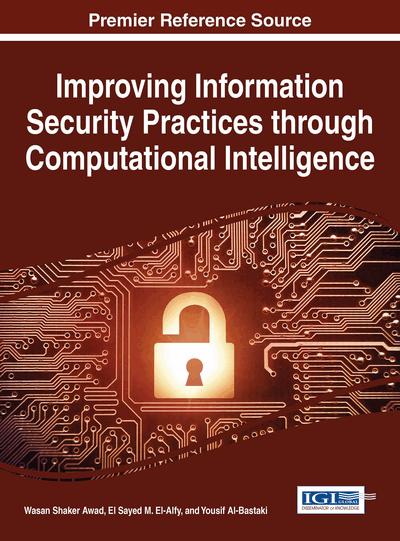# Computational Aspects of Lattice-Based Cryptography on Graphical Processing Unit

Sedat Akleylek (Ondokuz Mayis University, Turkey) and Zaliha Yuce Tok (Middle East Technical University, Turkey)
Copyright: © 2016 |Pages: 30
DOI: 10.4018/978-1-4666-9426-2.ch010
OnDemand PDF Download:
Available
\$37.50
No Current Special Offers

## Abstract

In this chapter, the aim is to discuss computational aspects of lattice-based cryptographic schemes focused on NTRU in view of the time complexity on a graphical processing unit (GPU). Polynomial multiplication algorithms, having a very important role in lattice-based cryptographic schemes, are implemented on the GPU using the compute unified device architecture (CUDA) platform. They are implemented in both serial and parallel way. Compact and efficient implementation architectures of polynomial multiplication for lattice-based cryptographic schemes are presented for the quotient ring both Zp [x]/(xn-1) and Zp [x]/(xn+1), where p is a prime number. Then, by using these implementations the NTRUEncrypt and signature scheme working over Zp [x]/(xn+1) are implemented on the GPU using CUDA platform. Implementation details are also discussed.
Chapter Preview
Top

## Introduction

Immediately after Shor proposed a polynomial time algorithm to solve integer factorization and discrete logarithm problem on a quantum computer (Shor, 1997), the demand to post-quantum cryptographic schemes started to increase. After this proposal, RSA, El-Gamal cryptosystem, elliptic curve based schemes became unreliable which causes a high demand for secure cryptographic protocols. These advancements also raise the importance of the studies on finding alternative systems that have efficient implementations on software/hardware platforms which are resistant to quantum attacks.

Post-quantum cryptographic schemes refer to the algorithms that are resistant to quantum attacks. These are the alternatives of public key cryptographic schemes such as RSA, DLP-based and elliptic curve based schemes. These schemes have been proposed for public key encryption, signature schemes and hash functions. Post-quantum cryptographic schemes can be classified as: code-based, hash-based, multivariate and lattice-based cryptography (Bernstein et al., 2009). The main problem in most of the post-quantum cryptographic schemes (code-based, multivariate and lattice-based cryptography) is the large key sizes. In hash-based schemes key sizes are relatively small such as 368-bit for 80-bit security. Due to large key sizes, in some cases they are not suitable for embedded devices such as smart cards, FPGAs. Another concern is the multiplication operation must be efficiently implemented in lattice-based cryptographic schemes since it is the most frequently used arithmetic operation.

Lattice-based cryptographic schemes are one of the most widely studied post-quantum cryptographic protocols. The security of these schemes depends on the hardness of lattice problems under some parameters (Bernstein et al., 2009). For several years lattice-based cryptographic schemes have only been considered secure for large system parameters causing inefficient implementations. Therefore, it’s thought that they were not practical. In 1998, NTRU cryptosystem was proposed as a public key cryptographic scheme over polynomial rings using the computational properties of hard problems over lattices as an alternative to factorization or discrete logarithm problem based schemes (Hoffstein et al., 1998). Standardization of the NTRU is drafted in IEEE P1363 (IEEE, 2008) still in progress and commercialized by Security Innovation (Security Innovation, 2014). After this draft was published, the progress has shown that the design has robustness against different kind of attacks. Its encryption process is almost 10 times faster and decryption processes is almost 100 times faster than RSA for the 1024-bit security level. Furthermore, there is no evidence that it’s vulnerable to practical or quantum attacks (Steinfeld, 2014). In this chapter, we focus on the arithmetic over the quotient ringused in NTRU schemes and alsoused in a signature scheme (Güneysu et al., 2012). The quotient ringcan work with Fast Fourier Transform-based multiplications resulting in a more efficient scheme. This is why this quotient ring is preferred in most of the recent ring-based cryptographic schemes (Banerjee et al. 2012; Lyubashevsky et al., 2008; Lyubashevsky et al., 2013).

## Complete Chapter List

Search this Book:
Reset